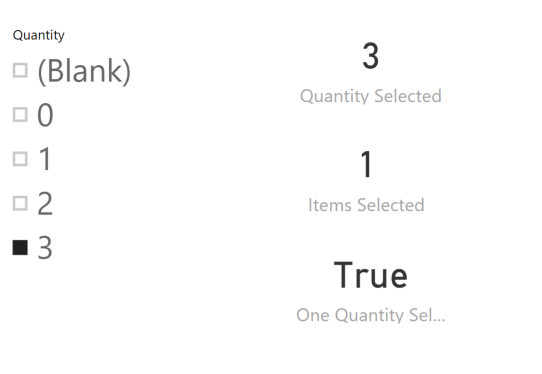I recently stumbled across a strange behavior of HASONEVALUE, which also impacts the SELECTEDVALUE function. When the only selected item in a column is a numeric value 0 and the column also contains a blank value, there is a bug in the current implementation that does not identify such a selection as a single one. Consider the following screenshot, where the slicer Quantity shows four values: (blank), 0, 1, 2, and 3.The measures displayed in the report are the following ones, which have the expected behavior in the previous screenshot.

```Quantity Selected :=
SELECTEDVALUE ( Sales[Quantity] )

Items Selected :=
COUNTROWS ( VALUES ( Sales[Quantity] ) )

One Quantity Selected :=
HASONEVALUE ( Sales[Quantity] )
```

The measure Quantity Selected reports the correct value selected in the Quantity slicer, which is 3.
The Items Selected measure reports that there is only one value selected, and also the One Quantity Selected measure returns True. So far, so good. However, when you select the value 0 in the slicer, the current behavior of Power BI (November 2017) has a buggy behavior, as you can see in the following screenshot.

Even if you selected only one item, the Items Selected measure reports two values selected in the current filter context. This happens because the current implementation considers the value 0 and (blank) as identical. In DAX, a blank value is automatically converted to 0 in case there is a comparison or an arithmetical operation. However, when applied to the slicer in Power BI, this result in the strange behavior shown in the previous picture.

In practice, this is what is happening. The following DAX query is an example of how the slicer applies a filter to the Items Selected measure:

```EVALUATE
CALCULATETABLE (
ROW (
"x",
COUNTROWS ( VALUES ( Sales[Quantity] ) )
),
Sales[Quantity] = 0
)
```

The result of the previous query is 2. The condition “Sales[Quantity] = 0” is true for two items, (blank) and 0. In practice, you cannot distinguish the previous query from the next one:

```EVALUATE
CALCULATETABLE(
ROW (
"x",
COUNTROWS ( VALUES ( Sales[Quantity] ) )
),
Sales[Quantity] = 0
|| ( ISBLANK ( Sales[Quantity] ) )
)
```

Because the VALUES function returns two rows, the HASONEVALUE function returns FALSE and the SELECTEDVALUE assumes that a multiple selection exists returning (blank).

If you want to get 1 as a result, you should write the following DAX query:

```EVALUATE
CALCULATETABLE (
ROW (
"x",
COUNTROWS ( VALUES ( Sales[Quantity] ) )
),
Sales[Quantity] = 0
&& NOT ( ISBLANK ( Sales[Quantity] ) )
)
```

This query returns 1 as a result.

Just to recap: selecting 0 in a slicer is like selecting both (blank) and 0. This behavior could change in the future, because it is considered a bug from usability point of view. If your report relies on this behavior, you should select both 0 and (blank) to make sure it will not break in the future.

If you need to identify the selection of 0 as a single selection, then you have a serious issue in case the same column also has (blank), because there is no way to recognize that the user selected only 0 and not (blank).

Strange behavior of HASONEVALUE and SELECTEDVALUE
containing the examples shown in this post.

HASONEVALUEReturns true when there’s only one value in the specified column.

`HASONEVALUE ( <ColumnName> )`

SELECTEDVALUEReturns the value when there’s only one value in the specified column, otherwise returns the alternate result.

`SELECTEDVALUE ( <ColumnName> [, <AlternateResult>] )`

VALUESWhen a column name is given, returns a single-column table of unique values. When a table name is given, returns a table with the same columns and all the rows of the table (including duplicates) with the additional blank row caused by an invalid relationship if present.

`VALUES ( <TableNameOrColumnName> )`